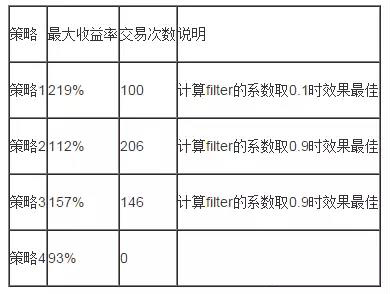理解AMA自适应移动平均线，并附策略源码（2）

Filter =percentage  * stdev( ama[i] –ama[i-1], n)

• Ama[i] – ama[i-1]> filter  or  Ama[i] – ama[i-2]> filter  买入
• Ama[i] – ama[i-1]< -filter  or  Ama[i] – ama[i-2]< -filter 卖出

• Filter =percentage * stdev( ma10[i] – ma10[i-1], 20) , 系数取值同上
• Close – ma10 > filter      买入
• Close – ma10 < -filter     卖出

• Filter =percentage * stdev( ama[i] – ama[i-1], n) , 系数取值同上
• Close – ama > filter      买入
• Close – ama < -filter     卖出DIRECTION:=CLOSE-REF(CLOSE,10);

VOLATILITY:=SUM(ABS((CLOSE-REF(CLOSE,1))),10);

ER:=ABS(DIRECTION/VOLATILITY);

FASTSC:=2/(2 + 1);

SLOWSC:=2/(30 + 1);

SSC:=ER*(FASTSC-SLOWSC)+SLOWSC;

cxh99.com:c,nodraw;

CONSTANT:=SSC*SSC;

AMAHIGH:=REF(EMA(HIGH,100),1)+CONSTANT*(HIGH- EMA(HIGH,100));

AMACLOSE:=REF(EMA(CLOSE,100),1)+CONSTANT*(CLOSE- REF(EMA(CLOSE,100),1));

AMALOW:=REF(EMA(LOW,100),1)+CONSTANT*(LOW- EMA(LOW,100));

REF(LOW>AMAHIGH,1),BK;

REF(CLOSE<AMACLOSE OR CLOSE<=0.995*BKPRICE,1),SP;

REF(HIGH<AMALOW,1),SK;

REF(CLOSE>AMACLOSE OR CLOSE>=1.005*SKPRICE,1),BP;

AUTOFILTER;

DIRECTION赋值:收盘价-10日前的收盘价

VOLATILITY赋值:(收盘价-昨收)的绝对值的10日累和

ER赋值:DIRECTION/VOLATILITY的绝对值

FASTSC赋值:2/(2 + 1)

SLOWSC赋值:2/(30 + 1)

SSC赋值:ER*(FASTSC-SLOWSC)+SLOWSC

CONSTANT赋值:SSC*SSC

AMAHIGH赋值:昨日最高价的100日指数移动平均+CONSTANT*(最高价- 最高价的100日指数移动平均)

AMACLOSE赋值:昨日收盘价的100日指数移动平均+CONSTANT*(收盘价- 昨日收盘价的100日指数移动平均)

AMALOW赋值:昨日最低价的100日指数移动平均+CONSTANT*(最低价- 最低价的100日指数移动平均)

AUTOFILTER